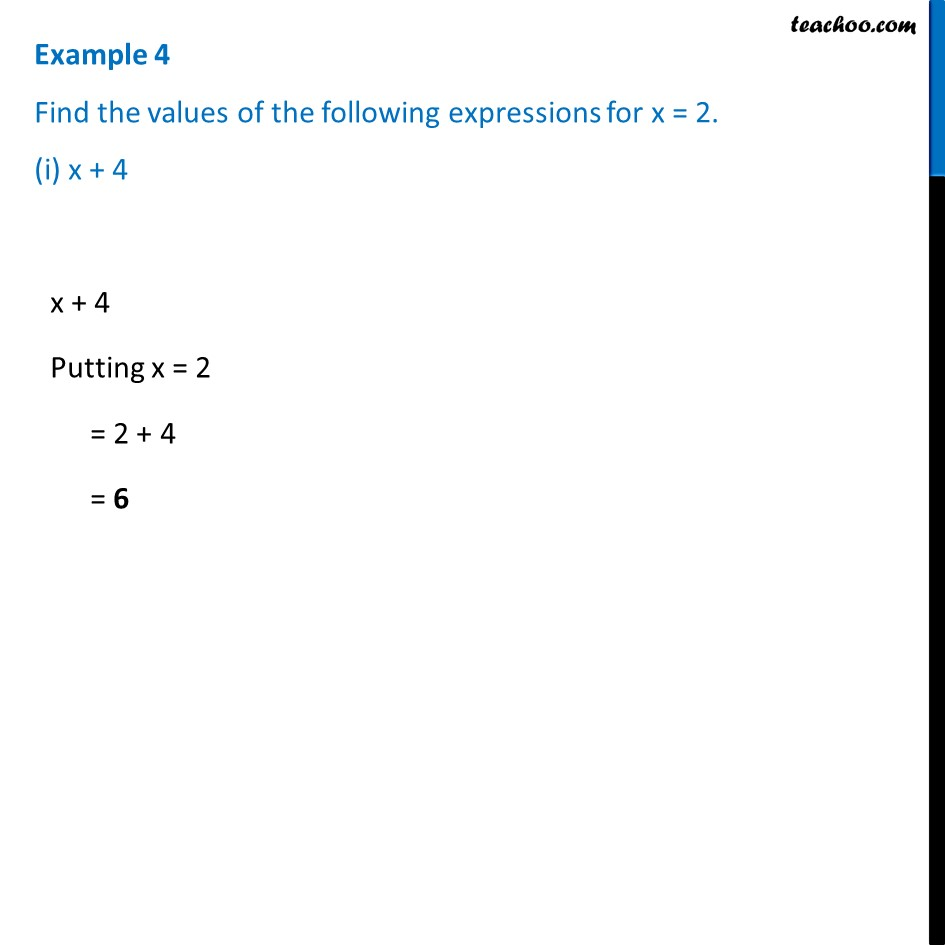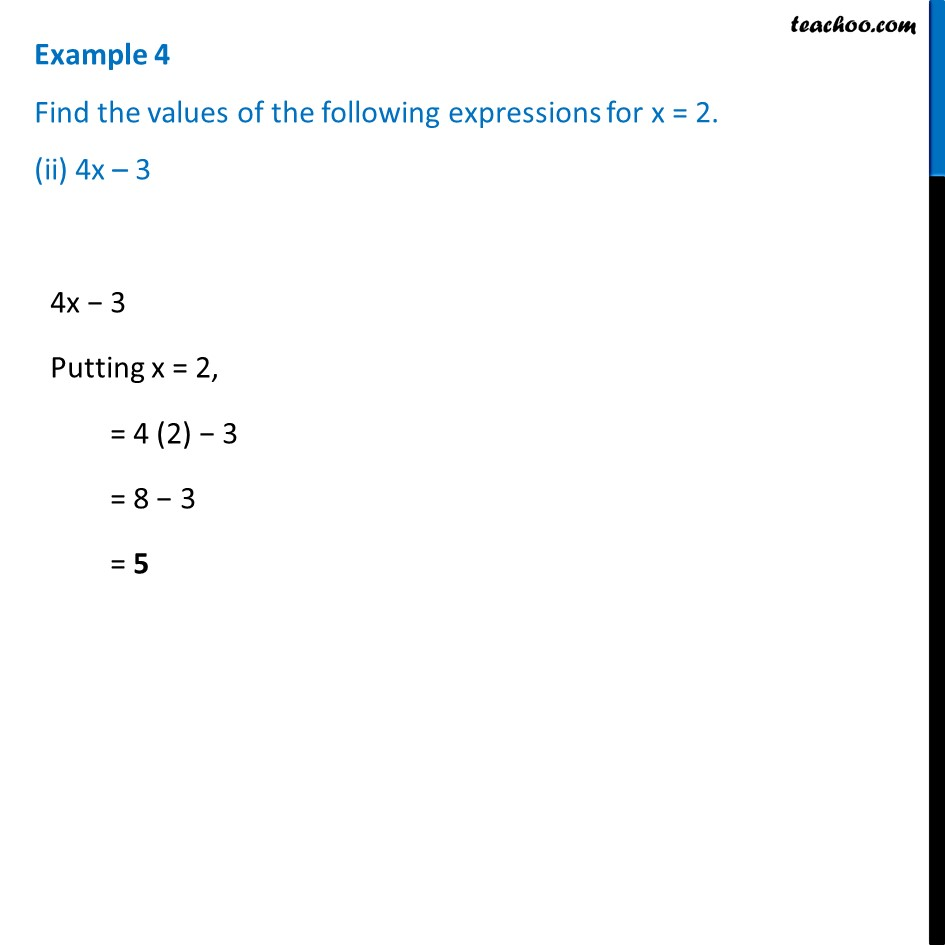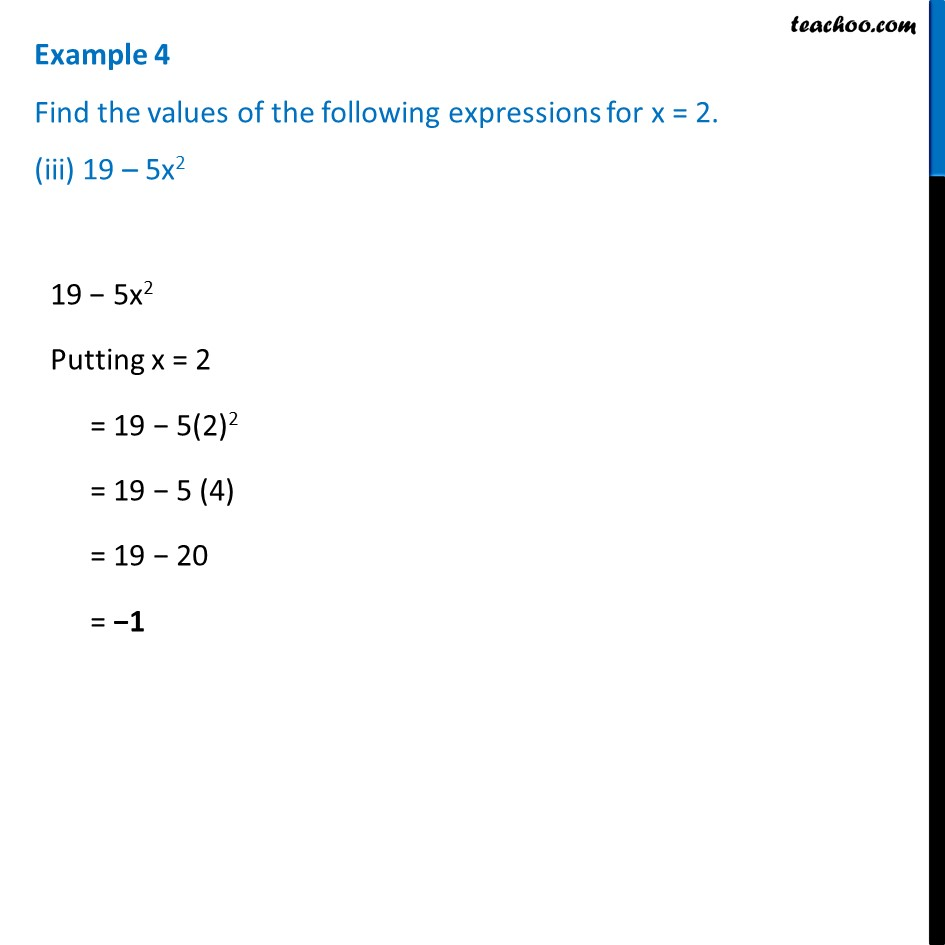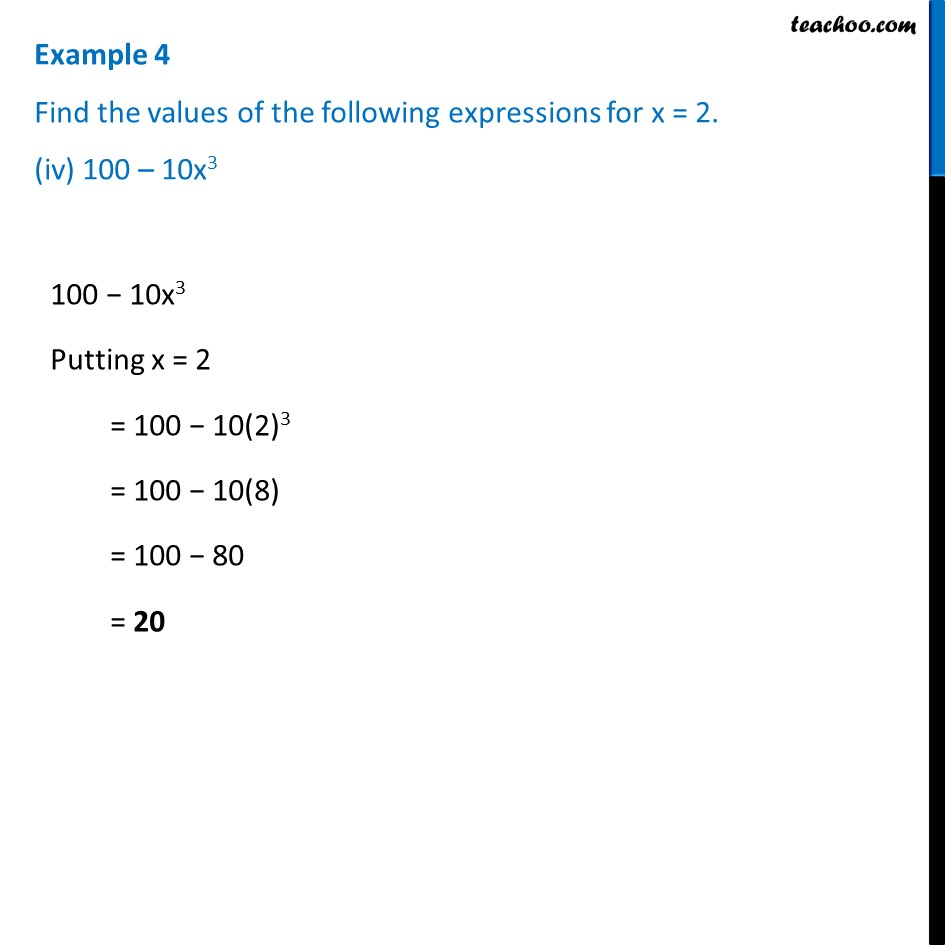Examples

Chapter 10 Class 7 Algebraic Expressions
Serial order wiseLearn in your speed, with individual attention - Teachoo Maths 1-on-1 Class

### Transcript

Example 4 Find the values of the following expressions for x = 2. (i) x + 4 x + 4 Putting x = 2 = 2 + 4 = 6 Example 4 Find the values of the following expressions for x = 2. (ii) 4x – 3 4x − 3 Putting x = 2, = 4 (2) − 3 = 8 − 3 = 5 Example 4 Find the values of the following expressions for x = 2. (iii) 19 – 5x219 − 5x2 Putting x = 2 = 19 − 5(2)2 = 19 − 5 (4) = 19 − 20 = −1 Example 4 Find the values of the following expressions for x = 2. (iv) 100 – 10x3 100 − 10x3 Putting x = 2 = 100 − 10(2)3 = 100 − 10(8) = 100 − 80 = 20